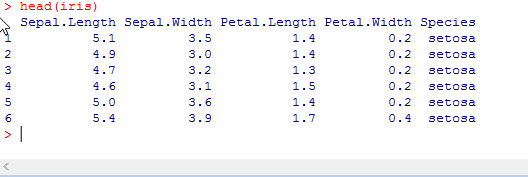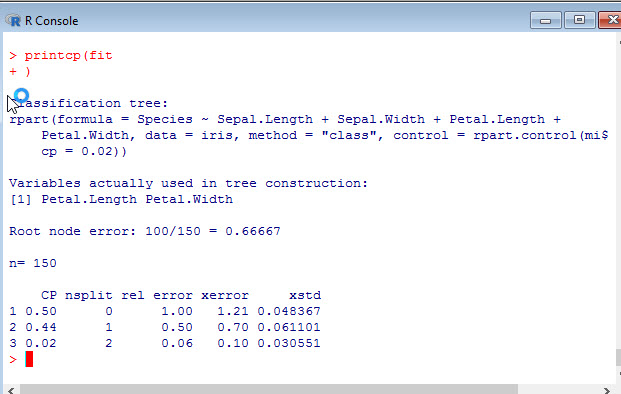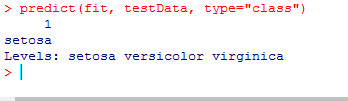# R: Decision Trees (Classification)

Decision Trees are popular supervised machine learning algorithms. You will often find the abbreviation CART when reading up on decision trees. CART stands for Classification and Regression Trees.

In this example we are going to create a Classification Tree. Meaning we are going to attempt to classify our data into one of the (three in this case) classes.

We are going to start by taking a look at the data. In this example we are going to be using the Iris data set native to R. This data set

`iris`As you can see, our data has 5 variables – Sepal.Length, Sepal.Width, Petal.Length, Petal.Width, and Species. The first 4 variables refer to measurements of flower parts and the species identifies which species of iris this flower represents. What we are going to attempt to do here is develop a predictive model that will allow us to identify the species of iris based on measurements.

The species we are trying to predict are setosa, virginica, and versicolor. These are our three classes we are trying to classify our data as.

In order to build our decision tree, first we need to install the correct package.

```install.packages("rpart")

library(rpart)```

Next we are going to create our tree. Since we want to predict Species – that will be the first element in our fit equation.

```fit <- rpart(Species ~ Sepal.Length + Sepal.Width + Petal.Length + Petal.Width,
method="class", iris)```

Now, let’s take a look at the tree.

```plot(fit)
text(fit)```

To understand what the output says, according to our model, if the Pedal.Length is < 2.45 then the flower is classified as setosa. If not, it goes to the next split – Petal Width. If < 1.75 then versicolor, else virginica.Now, we want to take a look at how good the model is.

`printcp(fit)`

I am not going to harp too much on the stats here, but lets look down at the table on the bottom. The first row has a CP = 0.50.  This means (approx) that the first split reduced the relative error by 0.5. You can see this in the rel error in the second row.

Now the 2nd row CP = 0.44, so the second split improved the rel error in the third row to 0.06.

Now personally, when just trying to get a quick overview of the goodness of the model, I look at the xerror (cross validation error) of the final row. 0.10 is a nice low number.Okay, now lets make a prediction. Start by creating some test data

```testData <-data.frame (Sepal.Length = 1, Sepal.Width = 4, Petal.Length =1.2,
+ Petal.Width=0.3)```

Now let’s predict

`predict(fit, testData, type="class")`

Here is the output:As you can see, the model predicted setosa. If you look back at the tree, you will see why.

Let’s do one more prediction

```newdata<-data.frame(Sepal.Length=c(3,8,7,5),
Sepal.Width=c(2,3,2,6),
Petal.Length=c(5.4,3.2,4.6,5.3),
Petal.Width=c(4,3,6,1.3))

predict (fit, newdata, type="class")```

Here is the outputThe model predicts 1,2,3 are virginica and 4 is versicolor.

Now go find some more data and try this out.

## 4 thoughts on “R: Decision Trees (Classification)”

1.olufemi

hi,
apart from rpart, which other algorithms are popularly used for classification?

1.Ben Larson

Some of the more popular classifiers would be Logistic regression – part of the glm() function , K nearest neighbor – knn(), and Naive Bayes naiveBayes(). You can also look at ensemble classifiers like Random Forests and Boosted Trees. I cover some of these in my Python tutorials where I explain how the algorithms work. I plan on making more R machine learning tutorials in the near future.

1.olufemi

Thanks a lot. i noticed that you mostly visually spot checked the accuracy of your models which i really like as it gives us a quick idea if we are on the right part or not. Do you have a tutorial or guidance on how to do this in R, possibly via a standard metric or so? Also , what about the caret package? When do you usually use that?

2.Laura

What is the difference of using the tree library